# US9176513B2 - High dynamic range exponential current generator with MOSFETs - Google Patents

## Info

Publication number
US9176513B2
US9176513B2 US14/243,741 US201414243741A US9176513B2 US 9176513 B2 US9176513 B2 US 9176513B2 US 201414243741 A US201414243741 A US 201414243741A US 9176513 B2 US9176513 B2 US 9176513B2
Authority
US
United States
Prior art keywords
current
current generator
circuit
aspect ratio
Prior art date
Legal status (The legal status is an assumption and is not a legal conclusion. Google has not performed a legal analysis and makes no representation as to the accuracy of the status listed.)
Active, expires
Application number
US14/243,741
Other versions
US20150286237A1 (en
Inventor
Munir A. Al-Absi
Karama M. Al-Tamimi
Current Assignee (The listed assignees may be inaccurate. Google has not performed a legal analysis and makes no representation or warranty as to the accuracy of the list.)
King Fahd University of Petroleum and Minerals
Original Assignee
King Fahd University of Petroleum and Minerals
Priority date (The priority date is an assumption and is not a legal conclusion. Google has not performed a legal analysis and makes no representation as to the accuracy of the date listed.)
Filing date
Publication date
Application filed by King Fahd University of Petroleum and Minerals filed Critical King Fahd University of Petroleum and Minerals
Priority to US14/243,741 priority Critical patent/US9176513B2/en
Assigned to KING FAHD UNIVERSITY OF PETROLEUM AND MINERALS reassignment KING FAHD UNIVERSITY OF PETROLEUM AND MINERALS ASSIGNMENT OF ASSIGNORS INTEREST (SEE DOCUMENT FOR DETAILS). Assignors: AL-ABSI, MUNIR A., DR., AL-TAMIMI, KARAMA M., MR.
Publication of US20150286237A1 publication Critical patent/US20150286237A1/en
Application granted granted Critical
Publication of US9176513B2 publication Critical patent/US9176513B2/en
Application status is Active legal-status Critical

• 230000036887 VSS Effects 0 claims 5
• 230000002457 bidirectional Effects 0 claims 2
• 238000004088 simulation Methods 0 description 7
• 238000000034 methods Methods 0 description 5
• 238000005516 engineering processes Methods 0 description 3
• 230000000875 corresponding Effects 0 description 2
• 230000000694 effects Effects 0 description 2
• 230000004044 response Effects 0 description 2
• 230000001052 transient Effects 0 description 2
• 238000004458 analytical methods Methods 0 description 1
• 230000003466 anti-cipated Effects 0 description 1
• 230000001976 improved Effects 0 description 1
• 239000011572 manganese Substances 0 description 1
• 230000035945 sensitivity Effects 0 description 1

## Images

•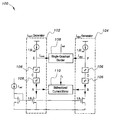•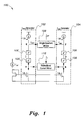•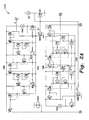•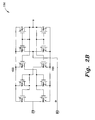•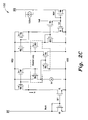•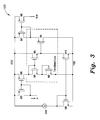•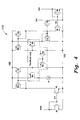•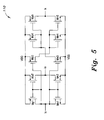•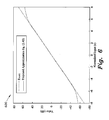•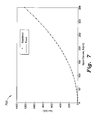•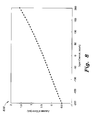•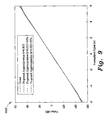•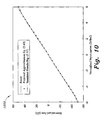## Classifications

• GPHYSICS
• G05CONTROLLING; REGULATING
• G05FSYSTEMS FOR REGULATING ELECTRIC OR MAGNETIC VARIABLES
• G05F3/00Non-retroactive systems for regulating electric variables by using an uncontrolled element, or an uncontrolled combination of elements, such element or such combination having self-regulating properties
• G05F3/02Regulating voltage or current
• G05F3/08Regulating voltage or current wherein the variable is dc
• G05F3/10Regulating voltage or current wherein the variable is dc using uncontrolled devices with non-linear characteristics
• G05F3/16Regulating voltage or current wherein the variable is dc using uncontrolled devices with non-linear characteristics being semiconductor devices
• GPHYSICS
• G05CONTROLLING; REGULATING
• G05FSYSTEMS FOR REGULATING ELECTRIC OR MAGNETIC VARIABLES
• G05F3/00Non-retroactive systems for regulating electric variables by using an uncontrolled element, or an uncontrolled combination of elements, such element or such combination having self-regulating properties
• G05F3/02Regulating voltage or current
• G05F3/08Regulating voltage or current wherein the variable is dc
• G05F3/10Regulating voltage or current wherein the variable is dc using uncontrolled devices with non-linear characteristics
• G05F3/16Regulating voltage or current wherein the variable is dc using uncontrolled devices with non-linear characteristics being semiconductor devices
• G05F3/20Regulating voltage or current wherein the variable is dc using uncontrolled devices with non-linear characteristics being semiconductor devices using diode- transistor combinations
• G05F3/26Current mirrors
• G05F3/262Current mirrors using field-effect transistors only

## Abstract

The high dynamic range exponential current generator produces an output waveform (current/voltage) which is an exponential function of the input waveform (current/voltage). The exponential characteristics are obtained in BiCMOS or Bipolar technologies using the intrinsic characteristics (IC/VBE) of the bipolar transistors. The high dynamic range exponential current generator is biased in weak inversion region. MOSFETs biased in weak inversion region are used not to utilize the inherent exponential (IDS/VGS) relationship but to simply implement x2 and x4 terms using translinear loops. The term x4 is realized by two cascaded squaring units. The approximation equation used is
$ⅇ x ≅ 0.025 + ( 1 + 0.125 ⁢ x ) 4 0.025 + ( 1 - 0.125 ⁢ x ) 4 .$

## Description

BACKGROUND OF THE INVENTION

1. Field of the Invention

The present invention relates to exponential generator circuits, and particularly to a high dynamic range exponential current generator utilizing MOSFETS operating in the weak inversion mode.

2. Description of the Related Art

An exponential function generator produces an output waveform (current/voltage) which is an exponential function of the input waveform (current/voltage). The exponential characteristics can be easily obtained in BiCMOS or Bipolar technologies using the intrinsic characteristics (IC/VBE) of the bipolar transistors. Though, it is not easy to realize such function in CMOS technology because of the inherent square-law or linear characteristics of MOSFETs operating in the strong inversion region. So the widely used technique to implement analog exponential function circuits using MOSFETs in strong inversion is based on pseudo-approximations. To mathematically implement the exponential function by this method, different approximations have been already introduced; Taylor series 2nd order, Taylor series 4th order, Pseudo exponential, Pseudo-Taylor approximation, Modified Pseudo-Taylor approximation, additional approximations have been proposed.

A MOSFET device biased in weak inversion region is a well-known approach to introduce an exponential function due to the exponential relationship between IDS and VGS of MOSFET in weak inversion regime. Referring to IDS/Vgs relationship, the drain current of MOSFET in weak inversion region is given by:

$I DS = 2 ⁢ n ⁢ ⁢ μ n ⁢ C ox ⁢ W L ⁢ V T 2 ⁢ ⅇ ( V gs - V th nU T )$

Although the low VGS voltage makes this technique efficient in low voltage applications compared with approximations that use MOSFET in strong inversion regime but, obviously, the exponential relation between IDS and VGS is not perfect because it suffers from strong temperature dependency, threshold voltage variation effect and sensitivity against process variation. Therefore, it is highly preferred to design an exponential function generator that provides accurate and stable exponential function vs. temperature variation; provides a robust and efficient design versus the supply voltage variation; utilizes current-input current-output exponential generator thereby providing higher frequencies of operation and wider dynamic ranges and extended output range with minimum linearity error.

Thus, a high dynamic range exponential current generator with MOSFETs solving the aforementioned problems is desired.

SUMMARY OF THE INVENTION

The high dynamic range exponential current generator produces an output waveform (current/voltage) which is an exponential function of the input waveform (current/voltage). The exponential characteristics are obtained in BiCMOS or Bipolar technologies using the intrinsic characteristics (IC/VBE) of the bipolar transistors. The high dynamic range exponential current generator is biased in weak inversion region. MOSFETs biased in weak inversion region are used not to utilize the inherent exponential (IDS/VGS) relationship but to simply implement x2 and x4 terms using translinear loops. The term x4 is realized by two cascaded squaring units. The approximation equation used is

$ⅇ x ≅ 0.025 + ( 1 + 0.125 ⁢ x ) 4 0.025 + ( 1 - 0.125 ⁢ x ) 4 .$

These and other features of the present invention will become readily apparent upon further review of the following specification and drawings.

BRIEF DESCRIPTION OF THE DRAWINGS

FIG. 1 is a block diagram of the high dynamic range exponential current generator according to the present invention.

FIGS. 2A-2C is a circuit diagram of the high dynamic range exponential current generator according to the present invention.

FIG. 3 is a circuit diagram of a squaring unit of the high dynamic range exponential current generator according to the present invention.

FIG. 4 is a circuit diagram of a single quadrant divider of the high dynamic range exponential current generator according to the present invention.

FIG. 5 is a circuit diagram of a current mirror of the high dynamic range exponential current generator according to the present invention.

FIG. 6 is a plot showing exact vs. proposed exponential approximation according to the present invention.

FIG. 7 is a plot showing exact vs. simulation results of the SQ block of the high dynamic range exponential current generator according to the present invention.

FIG. 8 is a plot showing BDCM error of the high dynamic range exponential current generator according to the present invention.

FIG. 9 is a plot showing effect of mismatch in the current mirror of the high dynamic range exponential current generator according to the present invention.

FIG. 10 is a plot showing linear-in-dB characteristics of the high dynamic range exponential current generator according to the present invention.

Similar reference characters denote corresponding features consistently throughout the attached drawings.

DETAILED DESCRIPTION OF THE PREFERRED EMBODIMENTS

The high dynamic range exponential current generator produces an output waveform (current/voltage) which is an exponential function of the input waveform (current/voltage). The exponential characteristics are obtained in BiCMOS or Bipolar technologies using the intrinsic characteristics (IC/VBE) of the bipolar transistors. The high dynamic range exponential current generator is biased in weak inversion region. MOSFETs biased in weak inversion region are used to simply implement x2 and x4 terms using translinear loops. The term x4 is realized by two cascaded squaring units 106. The exponential function generator approximation equation used is characterized by the relation,

$ⅇ x ≅ 0.025 + ( 1 + 0.125 ⁢ x ) 4 0.025 + ( 1 - 0.125 ⁢ x ) 4 , ( 1 )$
and has a dynamic range of approximately 96 dB. Plot 700 of FIG. 7 shows exact vs. simulation results of the squaring unit 106.

The full block diagram of the present high dynamic range exponential current generator with MOSFETs 100 is shown in FIGS. 2A-2C. The number of transistors used in the overall circuit is 65 MOSFETs without any passive elements and all of them are stacked between ±0.75V voltage-supply.

The squaring unit 106 is shown in detail in FIG. 3. The voltage supply is ±0.75V and the aspect ratios of the transistors are illustrated in Table 1. The constant currents equal to 4 Iref and 1.6 Iref can be easily provided by a proper current source and current sink of current Iref; e.g. if the current Iref shown in FIG. 1 is set to be 25 nA, then the constant current 4 Iref flowing through M9 (shown in FIG. 3) will be 100 nA. With reference to the MOSFET circuitry of squaring unit 106, by applying Translinear Loop (TL) through transistors M1-M4 then,
V gs1 +V gs2 =V gs3 +V gs4,   (2)
where Vgs1, Vgs2, Vgs3 and Vgs4 are the gate-to-source voltages of M1, M2, M3 and M4 respectively. From equation (2), one obtains the following:
I 1 I 2 =I 3 I 4.   (3)
Since I1=I2=Ix, I3=4Iref and I4=Iout then the output current will be expressed as follows:

$I out = I x 2 4 ⁢ I ref . ( 4 )$
Equation (4) represents the current-mode squaring function. Since the squaring circuit 106 is a key block in the present current-mode exponential generator 100, the simulation results have been carried out to demonstrate the validity of the theory. The corresponding maximum error is 1.5% and the circuit is stable with temperature variation. Table 1 details the aspect ratios of the squaring unit.

TABLE 1 Aspect ratios of squaring unit       Transistor $Aspect ⁢ ⁢ Ratio W ⁡ ( μm ) L ⁡ ( μm )$       Ratio M1, M3  3.5/7  0.5 M2, M4 91.7/7 13.1 M5-M10   7/7 1

With respect to the current divider 108, as shown in FIG. 4, the transistors involved in dashed box Ma-Md forms a single-quadrant current divider where all transistors are operating in the sub-threshold region. Analysis of the Ma-Md loop results in:
V sga +V sgb =V sgc +V sgd,   (5)
I a I b =I c I d,   (6)
with Ia=Iw, Ib=0.125 Inum, Ic=0.125 Iden, and Id=Iout. Then the equation (6) becomes

$I out , Divider = I w ⁢ I num I den . ( 7 )$
The transistor ratios are shown in Table 2. The

$( W L ) j , l = 1 8 ⁢ ( W L ) i , k$
scale down the currents Inum and Iden so that transistors Mb (representing the dividend quantity) and Mc (representing the divisor quantity) can absorb this amount of current and as a result the quotient amount (represented by Md) can be improved in terms of accuracy. This implies that the aspect ratios of all the transistors involved in the translinear loop must be selected to meet the anticipated dynamic range of the input and output currents. Table 2 details the transistor dimensions of the single quadrant divider circuit 108.

TABLE 2 Transistor dimensions of the single quadrant divider circuit       Transistor $Aspect ⁢ ⁢ Ratio W ⁡ ( μm ) L ⁡ ( μm )$       Ratio Ma, Md 196/1.4  140 Mb, Md 175/1.4  125 Me-Mh 7/7 1 Mi, Mk 19.6/19.6 1 Mj, Ml 2.45/19.6 0.125 Mm-Mn 1/1 1

As shown in FIG. 5, the current mirror circuitry (CM) 110 has two output currents. If the input current is Ix then two copies of this current can obtained at the output, Ix and −Ix. The dimensions of CM 110 are listed in Table 3. The simulation results with ±0.75V voltage supply are shown to verify the functionality of the circuit. Plot 600 of FIG. 6 shows exact vs. the present exponential approximation. With respect to the DC transfer characteristics and transient response, the calculated error is very small, as shown in plot 800 of FIG. 8.

TABLE 3 Dimensions of CM       Transistor $Aspect ⁢ ⁢ Ratio W ⁡ ( μm ) L ⁡ ( μm )$       Ratio Mn1-Mn5   1/10 0.1  Mp1-Mp5 1.7/10 0.17

With reference to the present current mode exponential generator 100 as shown in FIG. 1, there are nodes A, B, C, D, E and F. The current flows through these nodes as follows:

$⁢ I A = 8 ⁢ I ref + I x = 8 ⁢ I ref ⁡ ( 1 + 0.125 ⁢ I x I ref ) ( 8 ) ⁢ I B = 8 ⁢ I ref - I x = 8 ⁢ I ref ⁡ ( 1 - 0.125 ⁢ I x I ref ) ( 9 ) ⁢ I C = ( 8 ⁢ I ref ) 2 ⁢ ( 1 + 0.125 ⁢ I x I ref ) 2 4 ⁢ I ref ( 10 ) ⁢ I D = ( 8 ⁢ I ref ) 2 ⁢ ( 1 - 0.125 ⁢ I x I ref ) 2 4 ⁢ I ref ( 11 ) ⁢ I E = ( 8 ⁢ I ref ) 4 ⁢ ( 1 + 0.125 ⁢ I x I ref ) 4 ( 4 ⁢ I ref ) 3 = 64 ⁢ I ref ⁡ ( 1 + 0.125 ⁢ I x I ref ) 4 ( 12 ) ⁢ I F = ( 8 ⁢ I ref ) 4 ⁢ ( 1 - 0.125 ⁢ I x I ref ) 4 ( 4 ⁢ I ref ) 3 = 64 ⁢ I ref ⁡ ( 1 - 0.125 ⁢ I x I ref ) 4 ( 13 ) I num = 1.6 ⁢ I ref + 64 ⁢ I ref ⁡ ( 1 + 0.125 ⁢ I x I ref ) 4 = 64 ⁢ I ref ⁡ [ 0.025 + ( 1 + 0.125 ⁢ I x I ref ) 4 ] ( 14 ) I den = 1.6 ⁢ I ref + 64 ⁢ I ref ⁡ ( 1 - 0.125 ⁢ I x I ref ) 4 = 64 ⁢ I ref ⁡ [ 0.025 + ( 1 - 0.125 ⁢ I x I ref ) 4 ] ( 15 )$
By recall of the equations, the output current of the present EXPFG will be

$I out = I w ⁢ I num I den = I w ⁢ { [ 0.025 + ( 1 + 0.125 ⁢ I x I ref ) 4 ] [ 0.025 + ( 1 - 0.125 ⁢ I x I ref ) 4 ] } ≅ I w ⁢ ⅇ ( I x I ref ) ⁢ ⁢ and , ( 16 ) ⁢ I out = I w ⁢ ⅇ ( I x I ref ) , ( 17 )$
where Iout is the output current, Ix is the input ac signal, Iref is a constant current and Iw is a DC component which can be used to scale the output signal. From equation (17), it is clear that the exponential current-mode generator can be realized and its output current can be adjusted by Iw. The full circuit of the present current-mode exponential function generator (EXPFG) 100 is shown in FIGS. 2A-2C.

Referring again to EXPFG 100 of FIG. 1, if the current mirror 1.6 Iref is not exact (i.e. it is equal to 1.6 Iref+ΔIref), then equations (14) to (16) can be reevaluated and the output current is expressed as:

$I out = I w ⁢ I num I den = I w ⁢ { [ k + ( 1 + 0.125 ⁢ I x I ref ) 4 ] [ k + ( 1 - 0.125 ⁢ I x I ref ) 4 ] } , ( 18 )$
where

$k = 0.025 + Δ ⁢ ⁢ I ref 64 ⁢ I den .$
Assuming that there is ±10% deviation from the exact value (0.025), the results shown in plot 900 of FIG. 9 demonstrate that the deviation is not significant.

The EXPFG Circuit 100 shown in detail in FIGS. 2A-2C is used to implement the present function and is verified by simulation in 0.35 μm CMOS process technology with supply voltage ±0.75V. The threshold voltage of PMOS and NMOS is 0.833V and 0.572V in this process technology. The Tanner simulation result is illustrated in plot 1000 of FIG. 10 where Iref equals to 25 nA. Thus the x-axis, 150 nA≦Ix≦150 nA, can be normalized as −6≦x≦6 for comparison purposes. The curve of the present function is very close to the ideal exponential function,

$I w ⁢ ⅇ ( I x 25 ⁢ ⁢ nA ) ,$
with a high output dynamic range, nearly 96 dB. The error between the present function and the ideal exponential function,

$I w ⁢ ⅇ ( I x 25 ⁢ ⁢ ⁢ nA ) ,$
is limited to ±0.5 dB when −137.5 nA≦Ix≦137.5 nA.

The simulation of transient response has been carried out with sinusoidal input signal of frequency 5 kHz. With respect to the results of normalized output current Iout (dB) at −25° C., +25° C. and +75° C., as expected the input\output characteristics are roughly stable with temperature variation. The linearity error remains less than ±1.5 dB for the full scale of the input current range. The maximum deviation of the output current was about ±1.27 dB and is occurred for the normalized value

$I x I ref = 5.25 .$

It is to be understood that the present invention is not limited to the embodiments described above, but encompasses any and all embodiments within the scope of the following claims.

## Claims (4)

We claim:
1. A high dynamic range exponential current generator with MOSFETs, comprising:
a numerator current generator;
first and second numerator current generator squaring circuits connected in-line within the numerator current generator;
a denominator current generator;
first and second denominator current generator squaring circuits connected in-line within the denominator current generator;
a single quadrant divider circuit connected between output of the first numerator current generator squaring circuit and output of the first denominator current generator squaring circuit;
a bidirectional current mirror circuit connected between output of the second numerator current generator squaring circuit and output of the second denominator current generator squaring circuit;
numerator current generator and denominator current generator being biased in a weak inversion region that provides an approximation characterized by a relation,
$ⅇ x ≅ 0.025 + ( 1 + 0.125 ⁢ x ) 4 0.025 + ( 1 - 0.125 ⁢ x ) 4 ;$
and
wherein all components of the exponential current generator are MOSFETs.
2. The high dynamic range exponential current generator with MOSFETs according to claim 1, wherein each of the squaring circuits further comprises a squaring circuit translinear loop consisting of first, second, third, and fourth squaring circuit translinear loop transistors, aspect ratio of first and third squaring circuit translinear loop transistors being approximately 0.5, aspect ratio of second and fourth squaring circuit translinear loop transistors being approximately 13.1, aspect ratio of non-translinear loop portion of the squaring circuit being approximately 1.
3. The high dynamic range exponential current generator with MOSFETs according to claim 2, wherein the single quadrant divider circuit further comprises:
a single quadrant divider circuit translinear loop consisting of first, second, third, and fourth single quadrant divider circuit translinear loop transistors, aspect ratio of first and fourth single quadrant divider circuit translinear loop transistors being approximately 140, aspect ratio of second and fourth single quadrant divider circuit translinear loop transistors being approximately 125, aspect ratio of non-translinear loop portion of the single quadrant divider circuit being approximately 1;
first, second, third and fourth VDD rail connected transistors having an aspect ratio of 1;
a VSS rail connected numerator current input following transistor of the single quadrant divider circuit;
a VSS rail connected denominator current input following transistor of the single quadrant divider circuit;
aspect ratio of the VSS rail connected numerator current input following transistor of the single quadrant divider circuit/VSS rail connected denominator current input following transistor of the single quadrant divider circuit being approximately 0.125;
a numerator current input transistor;
a denominator current input transistor;
aspect ratio of the numerator current input transistor/denominator current input transistor being approximately 1; and
first and second single quadrant divider output current transistors having their gates connected together, drain of the first single quadrant divider output current transistor having its drain connected to drain of the fourth single quadrant divider translinear loop transistor, the current output being taken from drain of the second single quadrant divider output current transistor, aspect ratio of the first/second single quadrant divider output current transistors being approximately 1.
4. The high dynamic range exponential current generator with MOSFETs according to claim 3, wherein the bidirectional current mirror circuit further comprises:
VDD rail connected transistors each having an aspect ratio of approximately 0.1; and
VSS rail connected transistors each having an aspect ratio of approximately 0.17.
US14/243,741 2014-04-02 2014-04-02 High dynamic range exponential current generator with MOSFETs Active 2034-07-26 US9176513B2 (en)

## Priority Applications (1)

Application Number Priority Date Filing Date Title
US14/243,741 US9176513B2 (en) 2014-04-02 2014-04-02 High dynamic range exponential current generator with MOSFETs

## Applications Claiming Priority (1)

Application Number Priority Date Filing Date Title
US14/243,741 US9176513B2 (en) 2014-04-02 2014-04-02 High dynamic range exponential current generator with MOSFETs

## Publications (2)

Publication Number Publication Date
US20150286237A1 US20150286237A1 (en) 2015-10-08
US9176513B2 true US9176513B2 (en) 2015-11-03

# Family

## Family Applications (1)

Application Number Title Priority Date Filing Date
US14/243,741 Active 2034-07-26 US9176513B2 (en) 2014-04-02 2014-04-02 High dynamic range exponential current generator with MOSFETs

## Country Status (1)

US (1) US9176513B2 (en)

## Families Citing this family (1)

* Cited by examiner, † Cited by third party
Publication number Priority date Publication date Assignee Title
CN108563277A (en) * 2018-06-11 2018-09-21 北京工业大学 A kind of exponential waveform current generating circuit based on CMOS

## Citations (8)

* Cited by examiner, † Cited by third party
Publication number Priority date Publication date Assignee Title
US6744319B2 (en) 2001-12-13 2004-06-01 Hynix Semiconductor Inc. Exponential function generator embodied by using a CMOS process and variable gain amplifier employing the same
US6882185B1 (en) 1998-07-02 2005-04-19 Qualcomm, Incorporated Exponential current generator and method
US7180358B2 (en) 2003-12-26 2007-02-20 Electronics And Telecommunications Research Institute CMOS exponential function generating circuit with temperature compensation technique
US7514980B2 (en) 2005-06-23 2009-04-07 Samsung Electro-Mechanics Co., Ltd. Exponential function generator and variable gain amplifier using the same
US20100259317A1 (en) * 2009-04-14 2010-10-14 Chung Yuan Christian University High-output-impedance current mirror
US7979036B2 (en) 2004-12-30 2011-07-12 Agency For Science, Technology And Research Fully integrated ultra wideband transmitter circuits and systems
US20120081168A1 (en) * 2010-10-01 2012-04-05 Texas Instruments Incorporated A Delaware Corporation Implementing a piecewise-polynomial-continuous function in a translinear circuit
US8305134B2 (en) 2009-03-02 2012-11-06 Semiconductor Technology Academic Research Center Reference current source circuit provided with plural power source circuits having temperature characteristics

## Patent Citations (8)

* Cited by examiner, † Cited by third party
Publication number Priority date Publication date Assignee Title
US6882185B1 (en) 1998-07-02 2005-04-19 Qualcomm, Incorporated Exponential current generator and method
US6744319B2 (en) 2001-12-13 2004-06-01 Hynix Semiconductor Inc. Exponential function generator embodied by using a CMOS process and variable gain amplifier employing the same
US7180358B2 (en) 2003-12-26 2007-02-20 Electronics And Telecommunications Research Institute CMOS exponential function generating circuit with temperature compensation technique
US7979036B2 (en) 2004-12-30 2011-07-12 Agency For Science, Technology And Research Fully integrated ultra wideband transmitter circuits and systems
US7514980B2 (en) 2005-06-23 2009-04-07 Samsung Electro-Mechanics Co., Ltd. Exponential function generator and variable gain amplifier using the same
US8305134B2 (en) 2009-03-02 2012-11-06 Semiconductor Technology Academic Research Center Reference current source circuit provided with plural power source circuits having temperature characteristics
US20100259317A1 (en) * 2009-04-14 2010-10-14 Chung Yuan Christian University High-output-impedance current mirror
US20120081168A1 (en) * 2010-10-01 2012-04-05 Texas Instruments Incorporated A Delaware Corporation Implementing a piecewise-polynomial-continuous function in a translinear circuit

## Also Published As

Publication number Publication date
US20150286237A1 (en) 2015-10-08

## Similar Documents

Publication Publication Date Title
Croon et al. An easy-to-use mismatch model for the MOS transistor
JP3152922B2 (en) Current mirror circuit
US3152250A (en) Circuit for performing the combined functions of the extraction of roots, multiplicaton, and division
Ma et al. A novel 1.2–V 4.5-ppm/° C curvature-compensated CMOS bandgap reference
Magnelli et al. A 2.6 nW, 0.45 V temperature-compensated subthreshold CMOS voltage reference
Pierret et al. Simplified long-channel MOSFET theory
US20080180070A1 (en) Reference voltage generation circuit
Becker-Gomez et al. A low-supply-voltage CMOS sub-bandgap reference
Liu Low voltage CMOS four-quadrant multiplier
US20100060345A1 (en) Reference circuit for providing precision voltage and precision current
Zarebski et al. The electrothermal large-signal model of power MOS transistors for SPICE
US7208998B2 (en) Bias circuit for high-swing cascode current mirrors
Porret et al. A compact non-quasi-static extension of a charge-based MOS model
Hirose et al. A CMOS bandgap and sub-bandgap voltage reference circuits for nanowatt power LSIs
US8760143B2 (en) Reference current generation circuit
US3602843A (en) Electronic multiplication device and electrical energy measuring system using same
US6177788B1 (en) Nonlinear body effect compensated MOSFET voltage reference
US6819183B1 (en) Temperature and process compensation of MOSFET operating in sub-threshold mode
Dai et al. Threshold voltage based CMOS voltage reference
US3368066A (en) Fast multiplier employing fieldeffect transistors
US20090284304A1 (en) Circuit for generating a temperature-compensated voltage reference, in particular for applications with supply voltages lower than 1v
Long A radiation effects large signal equivalent circuit for MOS transistors
US20120319738A1 (en) Semiconductor integrated circuit device
US2976430A (en) Function generator circuits
US6323719B1 (en) Pseudo bipolar junction transistor

## Legal Events

Date Code Title Description
AS Assignment

Owner name: KING FAHD UNIVERSITY OF PETROLEUM AND MINERALS, SA

Free format text: ASSIGNMENT OF ASSIGNORS INTEREST;ASSIGNORS:AL-ABSI, MUNIR A., DR.;AL-TAMIMI, KARAMA M., MR.;REEL/FRAME:032587/0262

Effective date: 20140101

STCF Information on status: patent grant

Free format text: PATENTED CASE

MAFP Maintenance fee payment

Free format text: PAYMENT OF MAINTENANCE FEE, 4TH YR, SMALL ENTITY (ORIGINAL EVENT CODE: M2551); ENTITY STATUS OF PATENT OWNER: SMALL ENTITY

Year of fee payment: 4